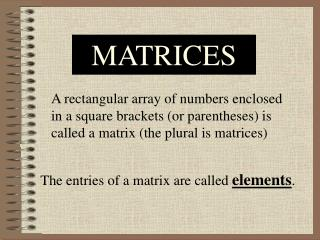# MATRICES - PowerPoint PPT PresentationDownload PresentationMATRICES

MATRICES
Download Presentation## MATRICES

- - - - - - - - - - - - - - - - - - - - - - - - - - - E N D - - - - - - - - - - - - - - - - - - - - - - - - - - -
##### Presentation Transcript

1. MATRICES A rectangular array of numbers enclosed in a square brackets (or parentheses) is called a matrix (the plural is matrices) The entries of a matrix are called elements.

2. Example #1 Consider the following two matrices. Is there any difference between them? Yes! M has 3 rows and 2 columns whereas N has 2 rows and 3 columns

3. Order of a matrix If a matrix has m rows and n columns, we say that it is a matrix of order m X n, read m by n. We also refer to it as an m X n matrix. The first number (m) refers to the number of rows and the second number (n) to the number of columns.

4. Example #2 Find the order of each of the following matrices: 2 X 3 1 X 4 3 X 1

5. Matrix Operations Now that we know the basic notions about matrices, we next consider how to perform operations with them. Addition We add matrices by adding corresponding elements.

6. Subtraction We subtract matrices by subtracting corresponding elements. Remark: To add or subtract matrices, they must have the same order. Multiplication by a number To multiply a matrix by a number, we multiply each element of the matrix by the number.

7. Example #3 Compute: where The matrices have the same order, so they can be added.

8. where

9. where Since the matrices have the same order, they can be added.

10. where Since the matrices don’t have the same order, we cannot compute their difference.

11. Equalityof matrices • Two matrices are equal if • they have the same order and • their corresponding elements are the same

12. Example #4 Find x and y if :

13. Example #5 The monthly expenditures (in dollars) for entertainment (E), food (F), and housing (H) per person under 25 and 25-34 years old are as show What would be the annual expenditures in the categories under 25 and 25-34?

14. To find the annual expenditures, we multiply the given matrix by 12 (since there are 12 months in a year) and obtain